Latest Teaching jobs   »   Data Handling : Download Mathematics Study...

# Data Handling : Download Mathematics Study Notes Free PDF For REET/UTET ExamMathematics is an equally important section for  REETMPTET & DSSSB Exams and has even more abundant importance in some other exams conducted by central or state govt. Generally, there are questions asked related to basic concepts and formulas of the Mensuration.

To let you make the most of Mathematics section, we are providing important facts related to the Unit Digit. At least 2-3 questions are asked from this topic in most of the teaching exams. We wish you all the best of luck to come over the fear of the Mathematics section.

How to Overcome Exam Fever, Especially When You Fear Maths

## Data Handling

Data handling comes under the branch of mathematics called statistics. Statistics is the science of drawing useful facts from some data. It includes systematic collection, presentation, and interpretation of the data with a view to find answers to some specific questions related to the data.

COLLECTION OF DATA

Mainly, there are two types of data:

(i) Primary data,

(ii) Secondary data.

Primary data: Data collected by an individual or his representative is called primary data. For example, if a person wants information about the number of school-going children or the number of family members of the employees of a firm, then either he himself or his representative gets the information from every individual of the firm. In this case, collected data is said to be primary data.

Secondary data: The data which is collected by someone else and used by the investigator for his requirements is called secondary data. The main sources of obtaining secondary data are:

1. Data published by the state agencies or central government agencies.
2. Reports published by some international organisations, etc.

Get free Study material for REET Exam

REPRESENTATION OF DATA

After collection and organisation of data in the tabular form, the next step is the representation of data. There are many ways to represent the data. Some of them are as follows:

Pictograph: In a pictograph, numerical data is represented by means of pictures.

The data can be represented as a pictograph as follows:

Scale: ? = 10 persons

 Males ? ? ? ? Females ? ? ? Children ? ? ? ? ? ?

Line graph: In a line graph, numerical data is represented by means of lines, by joining different points.

Example of line graph: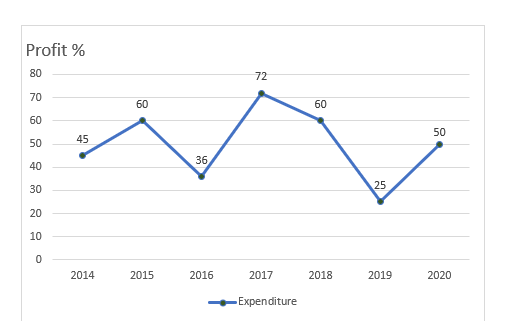Practice REET Previous Year Maths Quiz For REET Exam Here

Bar graph: In a bar graph, numerical data is represented by means of bars (rectangles of uniform width) with equal spacing between them. It is also called a bar chart.

Example of Bar graph: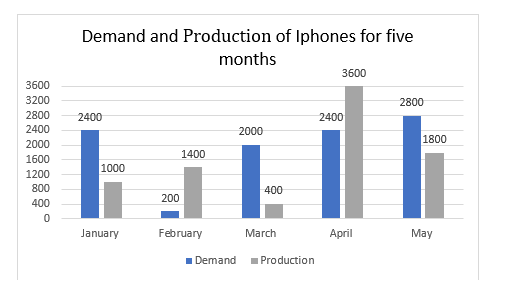Histogram: In a histogram, frequency distribution is represented by joining adjacent bars.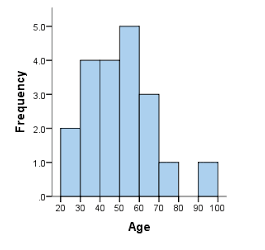Pie graph: In a pie graph, numerical data is represented in a circular form, i.e., by a circle. It is also called a pie chart or circle graph. In this graph, various components of data are expressed as a portion of 360°.

Example of pie graph or pie chart: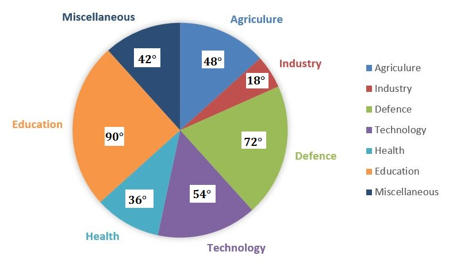Mathematics Study Notes For All Teaching Exams

Average

An average is a number that represents a group of numbers. The average represents the central value of a group of data. There are three types of averages:

(i) Arithmetic mean (or simply mean)

(ii) Median

(iii) Mode

Mean: The mean of a collection of given data is the sum of all the observations divided by the total number of observations.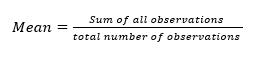Example:

A batsman scored the following runs in six different innings:

37, 40, 50, 49, 60, 58

Calculate the mean of the runs scored by him in an innings.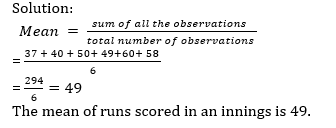Median: The median of a group of numbers is the number in the middle, when the numbers are arranged in an order. When the number of collections is odd, then the middle number is the median and when the number of collections is even, then the median is the average of the two middle numbers.

Example :

Find the median of the given data:

11, 16, 12, 15, 20, 17, 19

Solution: 11, 12, 15, 16, 17, 19, 20

Median = 16

Mode: The mode of a group of numbers is the number that appears most often.

Example: Find the mode, the following numbers are the runs score by a team in a match.

20, 30, 12 ,30 ,14, 76 ,82 ,20 ,64 ,20 ,7

Solution: The mode is 20, as it occurs 3 times

•रस - परिभाषा, भे�...
•Learning Disabilities : Math Dyslexia, D...
•Flander's Interaction Category System (F...
•Kothari Commission (1964-66) कोठ�...
•विशेषण- परिभाष�...
•Phases of Teaching - Stages of Teaching ...